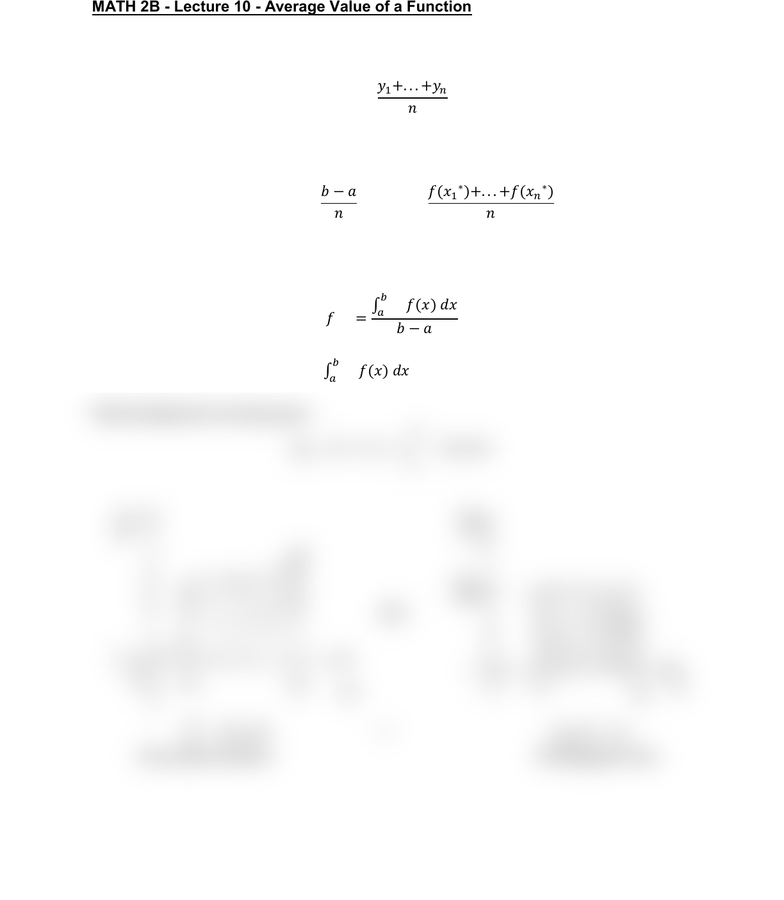Class Notes (1,100,000)
US (460,000)
UC-Irvine (10,000)
MATH (1,000)
MATH 2B (600)
Lecture 10

# MATH 2B Lecture Notes - Lecture 10: Riemann SumPremium

Department
Mathematics
Course Code
MATH 2B
Professor
ERJAEE, G.
Lecture
10

This preview shows half of the first page. to view the full 2 pages of the document.01/28/2019
MATH 2B - Lecture 10 - Average Value of a Function
Recall the usual meaning of average: if we have a collection of n values then
its average is

Now observe that a Riemann sum for a function f on an interval [a,b] is simply the
average value of the rectangle-heights , multiplied by the length of [a,b]:

 
This motivates us to define the notion of average for any integrable function.
The average value of a function f over [a,b] is:


We knew that the definite integral
 is the net area bounded by f(x) on [a,b].
Reformulating the formula gives:


 = 
Area under function Rectangular Area